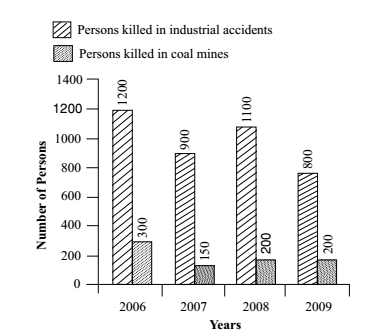## Bar graph

#### Data Interpretation

Direction: Study the bar diagram and answer questions based on it.1. The number of persons killed in coal mines in 2006 was what percent of those killed in industrial accidents in that year?

1.  Required percentage = The number of persons killed in coal mines in 2006 X 100% The number of persons killed in industrial accidents in 2006

##### Correct Option: B

As per given graph , we can see that
The number of persons killed in coal mines in 2006 = 300
The number of persons killed in industrial accidents in 2006 = 1200

 ∴ Required percentage = The number of persons killed in coal mines in 2006 X 100% The number of persons killed in industrial accidents in 2006

 ∴ Required percentage = 300 X 100% = 25% 1200

1. In which year, minimum number of persons killed in industrial accidents and coal mines together?

1. From the above given figure , the minimum number of persons killed in industrial accidents and coal mines are ( 800 + 200 ) = 1000 in 2009

##### Correct Option: D

From the above given figure , the minimum number of persons killed in industrial accidents and coal mines are ( 800 + 200 ) = 1000 in 2009
Hence the minimum number of persons were killed = 1000 (In 2009)

1. In which year, maximum number of persons were killed in industrial accidents other than those killed in coal mines?

1. From the above given figure , we can see that
The maximum number of persons killed in industrial accidents are 1200 in 2006

##### Correct Option: A

From the above given figure , we can see that
The maximum number of persons killed in industrial accidents are 1200 in 2006
Hence the maximum number of persons were killed in industrial accidents = 1200 (in 2006)

1. In which year, minimum number of persons were killed in coal mines other than those killed in industrial accidents?

1. From the given above figure , the minimum number of persons killed in coal mines are 150 in 2007

##### Correct Option: B

From the given above figure , the minimum number of persons killed in coal mines are 150 in 2007
Hence the minimum number of persons were killed in coal mines = 150 (in 2007)

1. In a year, on an average, how many persons were killed in industrial accidents and coal mines together?

1.  ∴ average = sum of the total persons killed in industrial accidents and coal mines total number of years

##### Correct Option: C

Total number of persons killed in industrial accidents and coal mines in
2006 = 1200 + 300 = 1500
2007 = 900 + 150 = 1050
2008 = 1100 + 200 = 1300
2009 = 800 + 200 = 1000
Total number of persons killed
= 1500 + 1050 + 1300 + 1000 = 4850

 ∴ average = sum of the total number of persons killed in industrial accidents and coal mines total number of years

 = 4850 = 1212.5 4